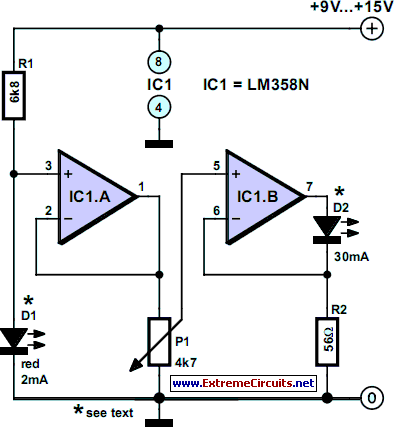# Learning Electronics

Learn to build electronic circuits

# 30mA LED Dimmer

If you’ve ever tried dimming a LED with a simple potentiometer, you know that the approach does not work very well. Just as with ordinary diodes, the voltage-current characteristic of LEDs is far from linear. The result - depending on the potentiometer setting the LED brightness will hardly change most of the time as the pot is turned and a sudden variation at the end. The best method to tackle this problem is to power the LED from a current source with zero to 100% adjustment range. The circuit shown here is an example. A low-current LED (D1) is used to generate a reference voltage that’s first buffered by one half of an LM358. The actual current source that powers the LED(s) is built around the second opamp in the chip.

Circuit diagram:30-mA LED Dimmer Circuit Diagram

The potentiometer allows the output current to be adjusted, with R2 acting as a current sense, the resistor dropping the same voltage as the one obtained from the pot. Using Ohm’s law we find that the maximum current through R2 amounts to about 29 mA (I LED = 1.6 V / 56 Ω). If necessary, the current may be adapted to suit other LED types, for example, 20 mA is obtained with R2 = 82 Ω and 10 mA at R2 = 150 Ω. It is also possible to connect several LEDs in series.

The total voltage available for the LEDs is determined by the voltage drop across series resistor and the opamp, and, of course, the supply voltage. In this way, the highest number of LEDs may be found from ULED, total = Ubatt – 5.1 V. In principle, it is possible to increase the supply voltage to 30 V in order to connect even more LEDs in series. This does, however, call for the value of series resistor R1 to be increased to prevent overloading the low-current LED used in the voltage reference. If you intend to experiment with larger numbers of LEDs (say, in arrays) then the maximum loading of the opamps becomes an issue. The DIP version of the LM358 may dissipate up to 830 mW. The power, P, is calculated from P = Ubatt – 1.6 – ULED,total × I LED,max.
Author: Eberhard Haug - Copyright: Elektor Electronics